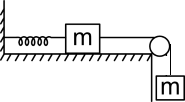Work Energy Theorem
Question

# Consider the system shown below, with two equal masses m and a spring with spring constant K. The coefficient of friction between the left mass and horizontal table is $\mathrm{\mu }=\frac{1}{4}$ , and the pulley is frictionless. The string connecting both the blocks is massless and inelastic. The system is held with the spring at its unstretched length and then released. The extension in spring when the masses come to momentary rest for the first time isModerate
Solution

## from work energy theorem,$\mathrm{w}=\mathrm{mgx}-\frac{1}{2}{\mathrm{kx}}^{2}-\mathrm{\mu mgx}=0$$\frac{2\mathrm{mg}}{\mathrm{k}}\left(1-\mathrm{\mu }\right)=\frac{3\mathrm{mg}}{2\mathrm{k}}$

Get Instant Solutions